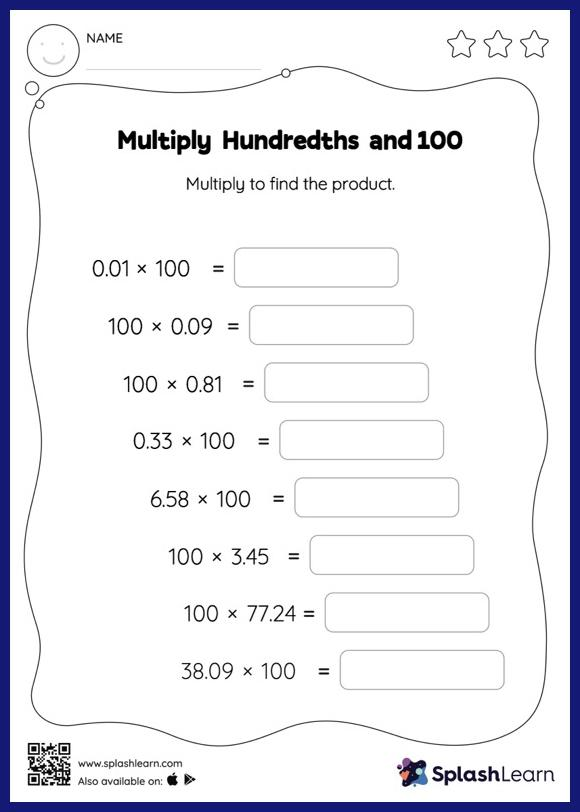# Multiply Hundredths and 100: Horizontal Multiplication Worksheet

Home > Multiply Hundredths and 100: Horizontal MultiplicationThe decimal point moves two places to the right when a decimal is multiplied by 100. Multiply hundredths and 100 worksheet requires students to apply this concept. As the worksheet uses a horizontal format, it allows for more creativity when it comes to how the student solves the problem. In contrast, in the vertical format, students usually employ the standard method to solve.